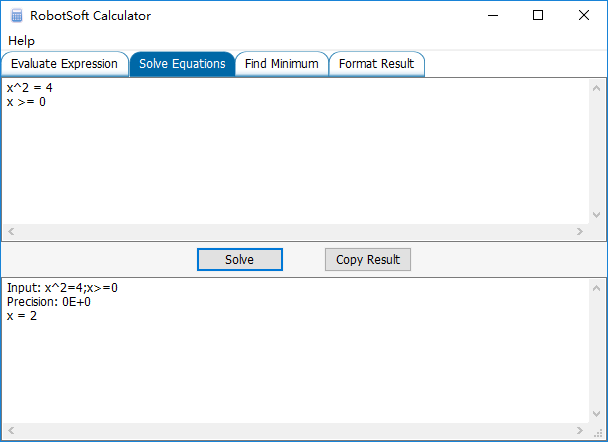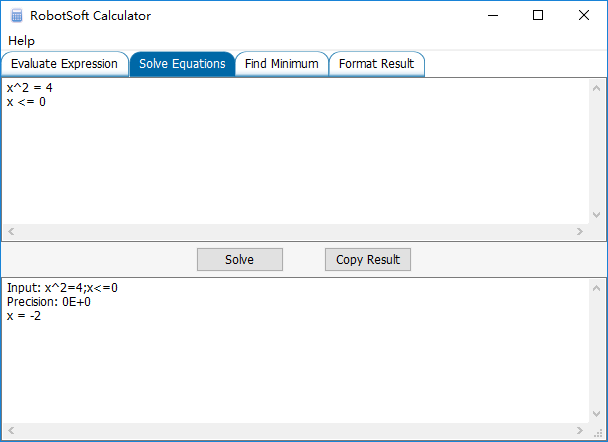## RobotSoft Calculator now supports Greater Equal and Less Equal command

Uncategorized Comments Off on RobotSoft Calculator now supports Greater Equal and Less Equal command
Nov 192016

The Solve Equations feature of RobotSoft Calculator now supports Greater Equal and Less Equal command. Different conditions may get different results.

Assume x >= 0:Assume x <= 0:## Solve Mathematical Equations Automatically

Uncategorized Comments Off on Solve Mathematical Equations Automatically
Nov 022014

Now Automatic Mouse and Keyboard has a new feature: Solve Equations Automatically. Call the function CreateSolver to create a solver, then call the solver’s method Solve. The method will return an array which contains the results.